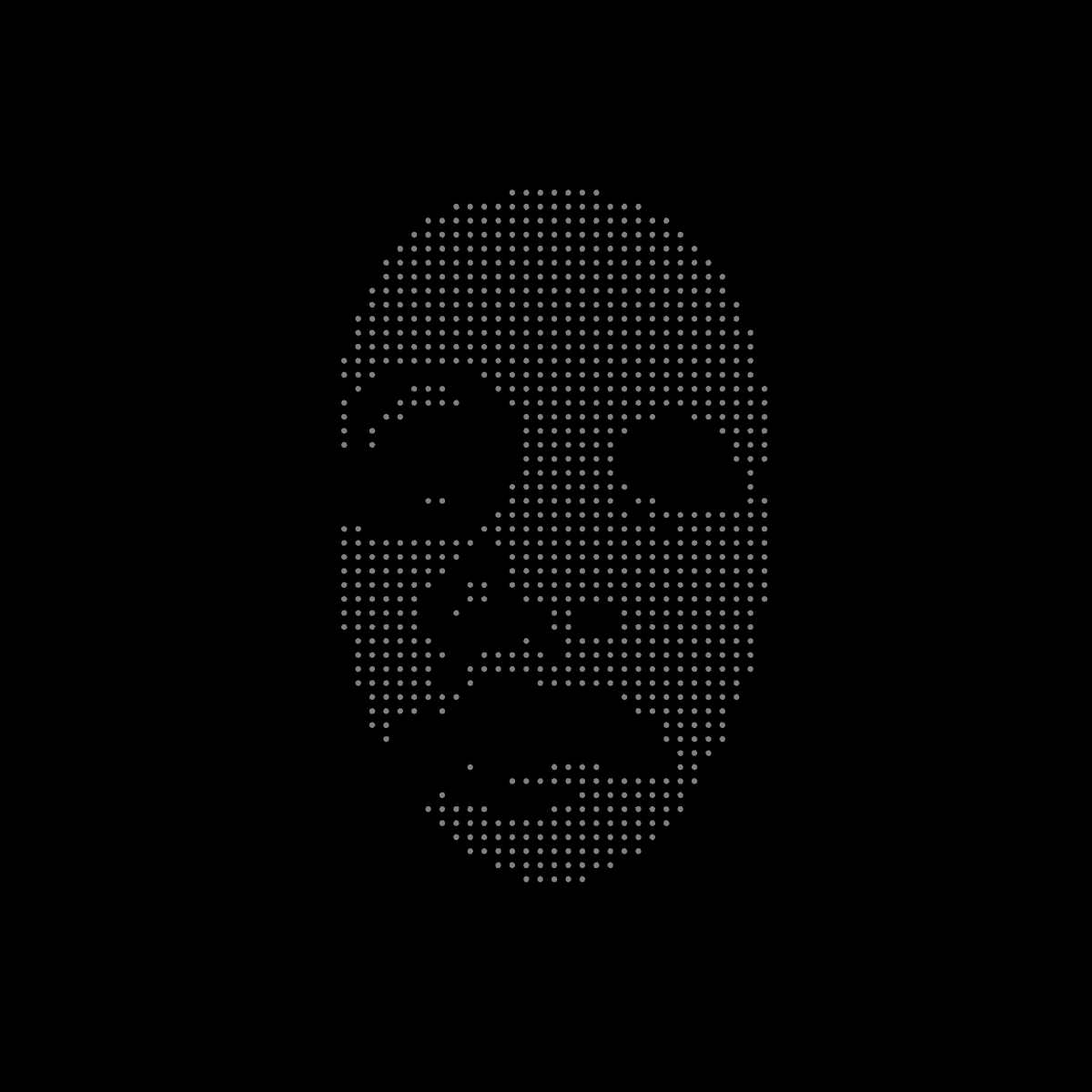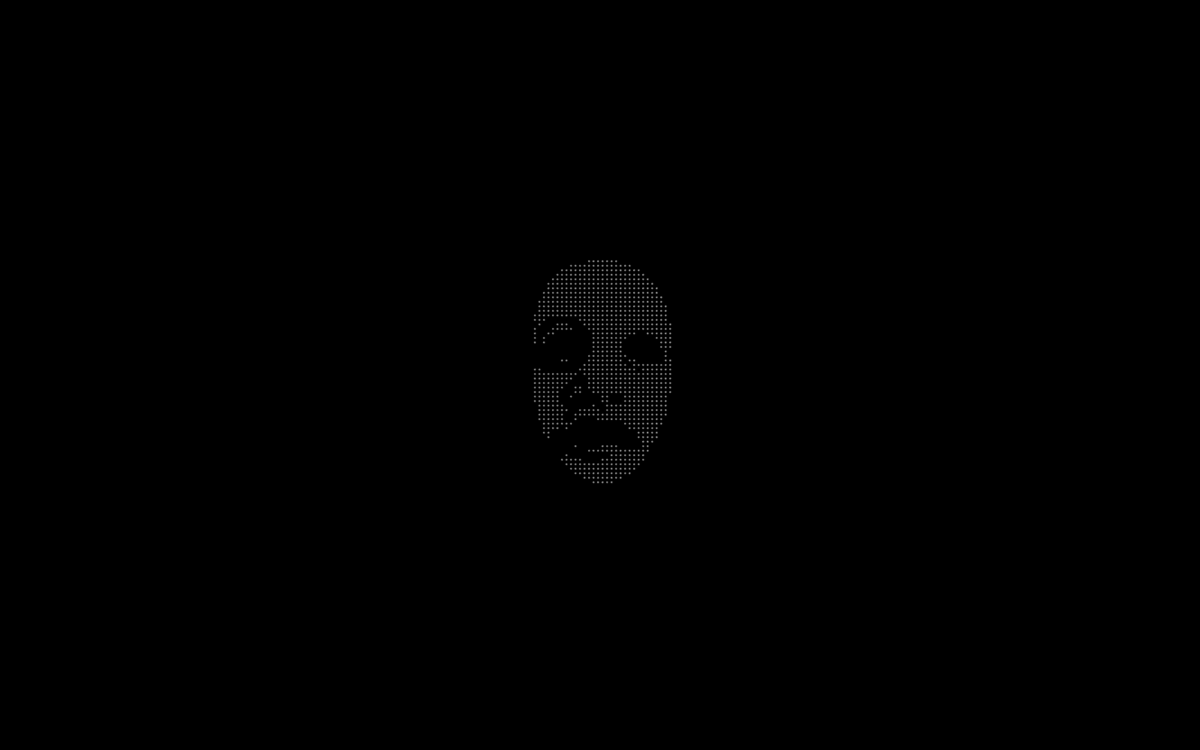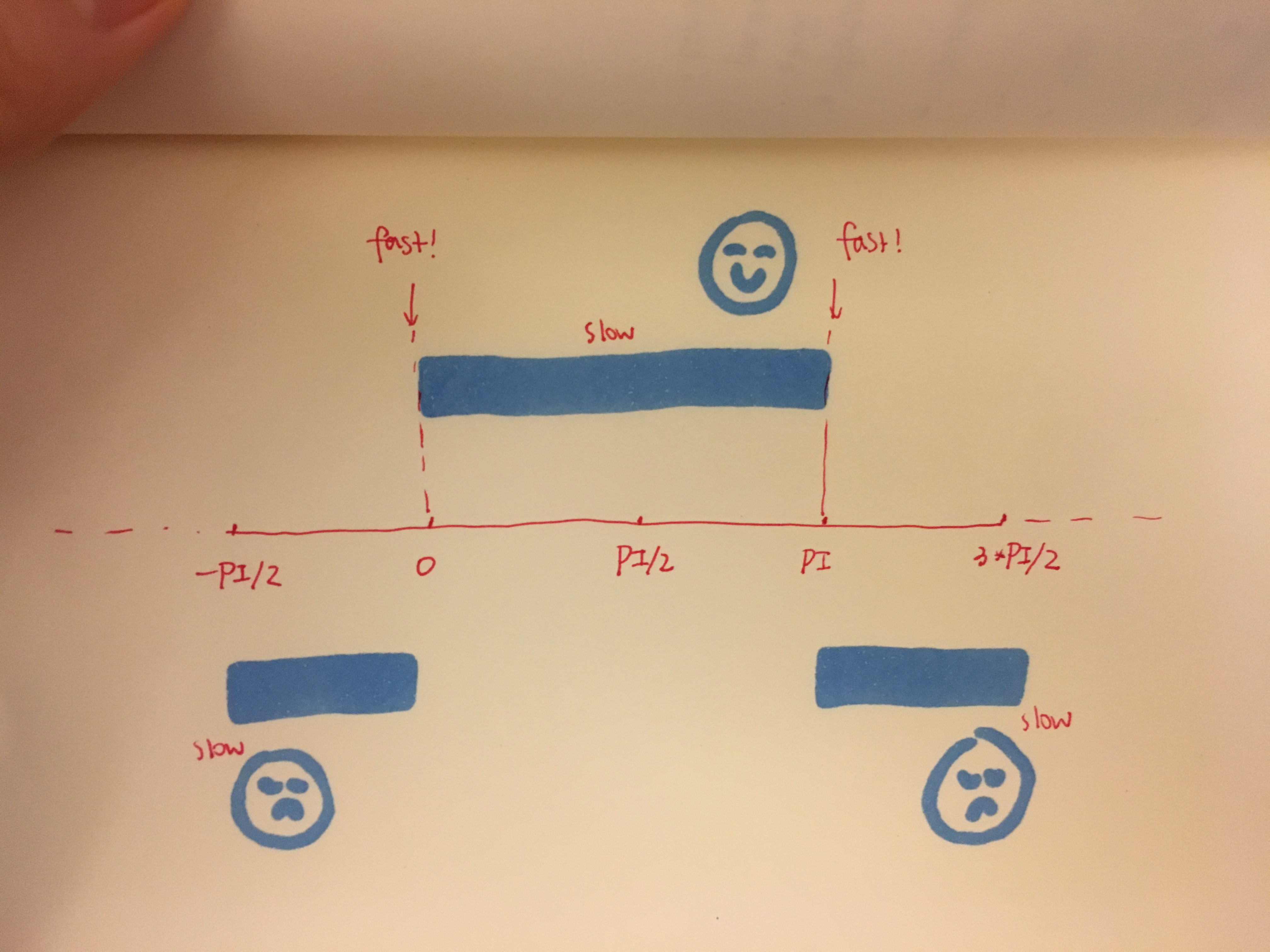# joxin-AnimatedLoopEmotions are a curious thing. They come and go in waves, often unexpected. Recently I read two very beautiful plays, Madea and Matsukaze. I learned about the masks used in ancient Greek theater and Japanese Noh theater to embody emotions and characters. They are abstract, dramatic representations of joy and suffering. I enjoy the simple and mysterious look, as well as the symbolic power.

Inspired by the masks, I decided to create a loop that signifies the cycle of emotions. Points vaguely define the visage of happiness and sadness. The smiling mask flips to the crying mask, and flips back. The points diffuse during the the flip, and then re-construct.

On one hand I want to strive for simplicity, but I also feel this overall graphics looks a bit “thin.” The concept is straight-forward, but there isn’t much to savor. I considered creating a grid of masks flipping together, but then I realized it wouldn’t really mean anything. There are other visual effects that I could add on top, but nothing feels like a plus with conceptual significance.

I thought this could be a fun screensaver, like this. Just playing with scale.The implementation goes like this: I scan the pixels of two high-contrast images of masks, and generate a point cloud that looks like a mask based on the brightness values. With the rotation of camera, I create the illusion of face flipping.```1 2 3 4 5 6 7 8 9 10 11 12 13 14 15 16 17 18 19 20 21 22 23 24 25 26 27 28 29 30 31 32 33 34 35 36 37 38 39 40 41 42 43 44 45 46 47 48 49 50 51 52 53 54 55 56 57 58 59 60 61 62 63 64 65 66 67 68 69 70 71 72 73 74 75 76 77 78 79 80 81 82 83 84 85 86 87 88 89 90 91 92 93 94 95 96 97 98 99 100 101 102 103 104 105 106 107 108 109 110 111 112 113 114 115 116 117 118 119 120 121 122 123 124 125 126 127 128 129 130 131 132 133 134 135 136 137 138 139 140 141 142 143 144 145 146 147 148 149 150 151 152 153 154 155 156 157 158 159 160 161 162 163 164 165 166 167 168 169 170 171 172 173 174 175 176 177 178 179 180 181 182 183 184 185 186 187 188 189 190 191 192 193 194 195 196 197 198 199 200 201 202 // This is a template for creating a looping animation in Processing/Java. // When you press the 'F' key, this program will export a series of images // into a "frames" directory located in its sketch folder. // These can then be combined into an animated gif. // Known to work with Processing 3.3.6 // Prof. Golan Levin, January 2018   // Thanks to help and feedback from Cameron Burgess, Faith Kim and Aman Tiwari &lt;3   //=================================================== // Global variables. String myNickname = "joxin"; int nFramesInLoop = 45; int nElapsedFrames; boolean bRecording;   PImage smile, cry; ArrayList dots; float scale = 1.8; float randomFactor = 3.5; float gaussianFactor = 13.0; int step = 16; float colorThreshold = 160;   //=================================================== void setup() { bRecording = false; nElapsedFrames = 0; size(600, 600, P3D); pixelDensity(2); background(0); smile = loadImage("smile.jpg"); cry = loadImage("cry.jpg"); smile.loadPixels(); cry.loadPixels(); dots = new ArrayList(); for (int x = 0; x &lt; cry.width; x += step) { for (int y = 0; y &lt; cry.height; y += step) { color c_cry = cry.get(x, y); color c_smile = smile.get(x, y); float x1 = 0; float y1 = 0; float x2 = 0; float y2 = 0; //float z = randomGaussian() * gaussianFactor - gaussianFactor/2; float z = randomGaussian() * gaussianFactor - gaussianFactor/2; if (red(c_cry) &gt; colorThreshold) { x1 = x/scale ;//+ random(-randomFactor, randomFactor); y1 = y/scale ;//+ random(-randomFactor, randomFactor); } if (red(c_smile) &gt; colorThreshold) { x2 = x/scale ;//+ random(-randomFactor, randomFactor); y2 = y/scale ;//+ random(-randomFactor, randomFactor); }   dots.add(new Dot(x1, y1, x2, y2, z, c_cry, c_smile)); } }   }   //=================================================== void keyPressed() { if ((key == 'f') || (key == 'F')) { bRecording = true; nElapsedFrames = 0; } }   //=================================================== void draw() {   // Compute a percentage (0...1) representing where we are in the loop. float percentCompleteFraction = 0; if (bRecording) { percentCompleteFraction = (float) nElapsedFrames / (float)nFramesInLoop; } else { percentCompleteFraction = (float) (frameCount % nFramesInLoop) / (float)nFramesInLoop; }   // Render the design, based on that percentage. renderMyDesign (percentCompleteFraction);   // If we're recording the output, save the frame to a file. if (bRecording) { saveFrame("frames/" + myNickname + "_frame_" + nf(nElapsedFrames, 4) + ".png"); nElapsedFrames++; if (nElapsedFrames &gt;= nFramesInLoop) { bRecording = false; } } }     void renderMyDesign (float percent) { background(0); lights();   float angle = 0; if (percent &lt;= 0.5) { float eased = function_DoubleExponentialSigmoid (percent*2, 0.7); angle = map(eased, 0, 1, -PI/2, PI/2);   } else { float eased = function_DoubleExponentialSigmoid (percent*2-1, 0.7); angle = map(eased, 0, 1, PI/2, 3*PI/2); }   camera(width/2+cos(angle)*width, height/2, sin(angle)*width, // eyeX, eyeY, eyeZ width/2, height/2, 0.0, // centerX, centerY, centerZ 0.0, 1.2, 0.0); // upX, upY, upZ}   for (int i = 0; i &lt; dots.size(); i++) { Dot d = dots.get(i); if (d != null) { d.show(percent); } }   }   class Dot { float x1, y1, x2, y2, z, c1, c2; Dot (float _x1, float _y1, float _x2, float _y2, float _z, float c_cry, float c_smile) { x1 = _x1; y1 = _y1; x2 = _x2; y2 = _y2; z = _z; c1 = c_cry; c2 = c_smile; }   void show(float percent) { float angleX, angleY, angleZ; if (percent &lt;= 0.5) { float easedX = function_DoubleExponentialSigmoid (percent*2, 0.7); float easedY = function_DoubleExponentialSigmoid (percent*2, 0.6); float easedZ = function_DoubleExponentialSigmoid (percent*2, 0.5); angleX = map(easedX, 0, 1, -PI/2, PI/2); angleY = map(easedY, 0, 1, -PI/2, PI/2); angleZ = map(easedZ, 0, 1, -PI/2, PI/2);   } else { float easedX = function_DoubleExponentialSigmoid (percent*2-1, 0.7); float easedY = function_DoubleExponentialSigmoid (percent*2-1, 0.6); float easedZ = function_DoubleExponentialSigmoid (percent*2-1, 0.5); angleX = map(easedX, 0, 1, PI/2, 3*PI/2); angleY = map(easedY, 0, 1, PI/2, 3*PI/2); angleZ = map(easedZ, 0, 1, PI/2, 3*PI/2); }   float maxScaleZ = 1.2; float maxScaleXY = 1; if (angleX &lt; 0 || angleX &gt; PI) { float expandFactor = 1; if (angleX &lt; 0) { expandFactor = map(abs(angleX - 0), 0, PI/2, maxScaleZ, 0); } else { expandFactor = map(abs(angleX - PI), 0, PI/2, maxScaleZ, 0); } float otherExpandFactor = map(expandFactor, maxScaleZ, 1, maxScaleXY, 1); float curZ = map(z, 0, 1, 0, expandFactor); if (x1 &gt; 0) { pushMatrix(); translate(width/2 - cry.width/2.0/scale*otherExpandFactor + x1*otherExpandFactor, height/2 - cry.height/2.0/scale*otherExpandFactor + y1*otherExpandFactor, curZ); noStroke(); sphere(1.8); popMatrix(); } } else { if (x2 &gt; 0) { float expandFactor = 1; if (angleX &lt; PI/2) { expandFactor = map(abs(angleX - 0), 0, PI/2, maxScaleZ, 0); } else { expandFactor = map(abs(angleX - PI), 0, PI/2, maxScaleZ, 0); } float otherExpandFactor = map(expandFactor, maxScaleZ, 1, maxScaleXY, 1); float curZ = map(z, 0, 1, 0, expandFactor); pushMatrix(); translate(width/2 - smile.width/2.0/scale*otherExpandFactor + x2*otherExpandFactor, height/2 - smile.height/2.0/scale*otherExpandFactor + y2*otherExpandFactor, curZ); noStroke(); sphere(1.8); popMatrix(); } } }   void setZ(float zz) { z = zz; } }   //=================================================== // Taken from https://github.com/golanlevin/Pattern_Master float function_DoubleExponentialSigmoid (float x, float a) { // functionName = "Double-Exponential Sigmoid";   float min_param_a = 0.0 + EPSILON; float max_param_a = 1.0 - EPSILON; a = constrain(a, min_param_a, max_param_a); a = 1-a;   float y = 0; if (x&lt;=0.5) { y = (pow(2.0*x, 1.0/a))/2.0; } else { y = 1.0 - (pow(2.0*(1.0-x), 1.0/a))/2.0; } return y; }```